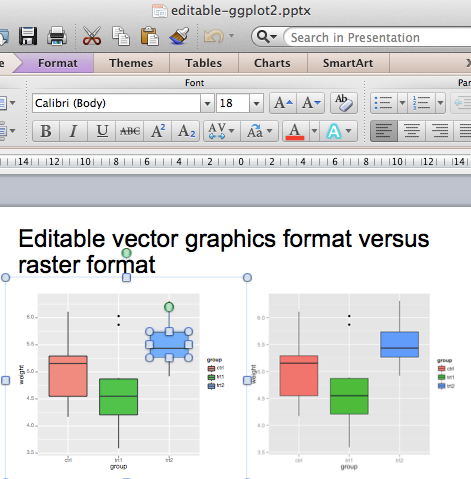# Create an editable graph from R software

In this article you’ll learn how to create an editable vector graphics from R software. This could be interesting in some cases and gives you the ability to edit your graphics from PowerPoint. You can change line types, colors, point shapes, etc, …If you want to export your plot from R to PowerPoint automatically, this is for you.

If you want to bring your ggplot2 charts to PowerPoint, then this guide is for you.

If you’re looking for an exact package to create an editable plot and to save it as a PowerPoint document, then you’ll love this tutorial.

If you’re a beginner in R programming, you’ll definitely learn something new that you can use if needed.

# What R package to use ?

Editable vector graphics can be created and saved in a PowerPoint document using ReporteRs package.

``````install.packages('ReporteRs') # Install

# Create editable plots

## Case of base graphs

The example below creates a PowerPoint document in your current working directory. The document contains one slide with 2 panels :

• The first panel contains an editable box plot
• The second panel contains a raster format

The PowerPoint document created by the R code below is available here : R software and ReporteRs package - create an editable base graph

``````library('ReporteRs')
# Create a new powerpoint document
doc <- pptx()
# Add a new slide into the ppt document
doc <- addSlide(doc, "Two Content" )
doc<- addTitle(doc, "Editable vector graphics format versus raster format" )
# A function for creating a box plot
boxplotFunc<- function(){
boxplot(len ~ dose, data = ToothGrowth,
col=2:4,main = "Guinea Pigs' Tooth Growth",
xlab = "Vitamin C dose mg",
ylab = "tooth length")
}
# Add an editable box plot
doc <- addPlot(doc, boxplotFunc, vector.graphic = TRUE )
# Add a raster box plot
doc <- addPlot(doc, boxplotFunc, vector.graphic = FALSE )
# write the document to a file
writeDoc(doc, file = "editable-graph.pptx")``````

Open the created PowerPoint and try to edit the first box plot by changing the fill colors, line types, etc…## Case of graphs generated using ggplot2

ggplot2 is a powerful R package, implemented by Hadley Wickham, for producing a visually appealing charts.

``````install.packages('ggplot2') # Install

Create an editable plot with ggplot2 :

``````library('ReporteRs')
library(ggplot2)
# Create a new powerpoint document
doc <- pptx()
# Add a new slide into the ppt document
doc <- addSlide(doc, "Two Content" )
doc<- addTitle(doc, "Editable vector graphics format versus raster format" )
# A function for creating a box plot
bp <- ggplot(data=PlantGrowth, aes(x=group, y=weight, fill=group))+
geom_boxplot()
# Add an editable box plot
doc <- addPlot(doc, function() print(bp), vector.graphic = TRUE )
# Add a raster box plot
doc <- addPlot(doc, function() print(bp), vector.graphic = FALSE )
# write the document to a file
writeDoc(doc, file = "editable-ggplot2.pptx")``````

The PowerPoint document created by the R code above is available here : R software and ReporteRs package - create an editable ggplot2# Infos

This analysis has been performed using R (ver. 3.1.0).

GitHub (David Gohel): ReporteRs